Home
Hostname: page-component-5c569c448b-nqqt6 Total loading time: 0.222 Render date: 2022-07-02T21:56:59.009Z Has data issue: true Feature Flags: { "shouldUseShareProductTool": true, "shouldUseHypothesis": true, "isUnsiloEnabled": true, "useRatesEcommerce": false, "useNewApi": true } hasContentIssue true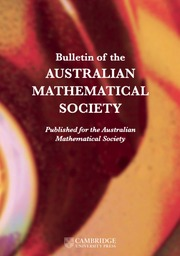Bulletin of the Australian Mathematical Society

## Abstract

We provide an easy method for the construction of characteristic polynomials of simple ordinary abelian varieties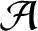${{\mathcal A}}$ of dimension g over a finite field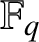${{\mathbb F}}_q$ , when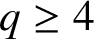$q\ge 4$ and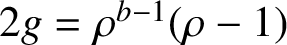$2g=\rho ^{b-1}(\rho -1)$ , for some prime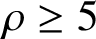$\rho \ge 5$ with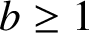$b\ge 1$ . Moreover, we show that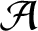${{\mathcal A}}$ is absolutely simple if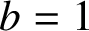$b=1$ and g is prime, but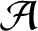${{\mathcal A}}$ is not absolutely simple for any prime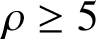$\rho \ge 5$ with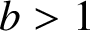$b>1$ .

## MSC classification

Type
Research Article
Information
Bulletin of the Australian Mathematical Society , December 2021 , pp. 391 - 397
© 2021 Australian Mathematical Publishing Association Inc.

## Access options

Get access to the full version of this content by using one of the access options below. (Log in options will check for institutional or personal access. Content may require purchase if you do not have access.)

## References

Burton, D., Elementary Number Theory, 7th edition (McGraw-Hill, New York, 2011).Google Scholar
Deligne, P., ‘La conjecture de Weil. I’, Inst. Hautes Études Sci. Publ. Math. 43 (1974), 273307.10.1007/BF02684373CrossRefGoogle Scholar
DiPippo, S. A. and Howe, E. W., ‘Real polynomials with all roots on the unit circle and abelian varieties over finite fields’, J. Number Theory 73(2) (1998), 426450.10.1006/jnth.1998.2302CrossRefGoogle Scholar
Dwork, B., ‘On the rationality of the zeta function of an algebraic variety’, Amer. J. Math. 82 (1960), 631648.10.2307/2372974CrossRefGoogle Scholar
Grothendieck, A., ‘Formule de Lefschetz et rationalité des fonctions$L$ ’, Séminaire Bourbaki, 9, Exp. No. 279 (Société Mathématique de France, Paris, 1995), 4155.Google Scholar
Guerrier, W. J., ‘The factorization of the cyclotomic polynomials mod$p$ ’, Amer. Math. Monthly 75 (1968) 46.CrossRefGoogle Scholar
Haloui, S., ‘The characteristic polynomials of abelian varieties of dimensions 3 over finite fields’, J. Number Theory 130(12) (2010), 27452752.10.1016/j.jnt.2010.06.008CrossRefGoogle Scholar
Haloui, S. and Singh, V., ‘The characteristic polynomials of abelian varieties of dimension 4 over finite fields’, Arithmetic, Geometry, Cryptography and Coding Theory, Contemporary Mathematics, 574 (American Mathematical Society, Providence, RI, 2012), 5968.CrossRefGoogle Scholar
Hartshorne, R., Algebraic Geometry, Graduate Texts in Mathematics, 52 (Springer-Verlag, New York, 1977).10.1007/978-1-4757-3849-0CrossRefGoogle Scholar
Honda, T., ‘Isogeny classes of abelian varieties over finite fields’, J. Math. Soc. Japan 20 (1968), 8395.10.2969/jmsj/02010083CrossRefGoogle Scholar
Howe, E. W., ‘Principally polarized ordinary abelian varieties over finite fields’, Trans. Amer. Math. Soc. 347 (1995), 23612401.CrossRefGoogle Scholar
Howe, E. W. and Zhu, H. J., ‘On the existence of absolutely simple abelian varieties of a given dimension over an arbitrary field’, J. Number Theory 92(1) (2002), 139163.10.1006/jnth.2001.2697CrossRefGoogle Scholar
Lakatos, P. and Losonczi, L., ‘Circular interlacing with reciprocal polynomials’, Math. Inequal. Appl. 10(4) (2007), 761769.Google Scholar
Lehmer, D. H., ‘Quasi-cyclotomic polynomials’, Amer. Math. Monthly 39(7) (1932), 383389.10.1080/00029890.1932.11987335CrossRefGoogle Scholar
Maisner, D. and Nart, E., ‘Abelian surfaces over finite fields as Jacobians’, with an appendix by Howe, Everett W., Experiment. Math. 11(3) (2002), 321337.10.1080/10586458.2002.10504478CrossRefGoogle Scholar
Rück, H., ‘Abelian surfaces and Jacobian varieties over finite fields’, Compositio Math. 76(3) (1990), 351366.Google Scholar
Singh, V., McGuire, G. and Zaytsev, A., ‘Classification of characteristic polynomials of simple supersingular abelian varieties over finite fields’, Funct. Approx. Comment. Math. 51(2) (2014), 415436.Google Scholar
Tate, J., ‘Endomorphisms of abelian varieties over finite fields’, Invent. Math. 2 (1966) 134144.CrossRefGoogle Scholar
Waterhouse, W. C., ‘Abelian varieties over finite fields’, Ann. Sci. École Norm. Sup. (4) 2 (1969), 521560.CrossRefGoogle Scholar
Waterhouse, W. C. and Milne, J. S., ‘Abelian varieties over finite fields’, Proc. Sympos. Pure Math. 20 (1971), 5364.10.1090/pspum/020/0314847CrossRefGoogle Scholar
Weil, A., ‘Numbers of solutions of equations in finite fields’, Bull. Amer. Math. Soc. 55 (1949), 497508 10.1090/S0002-9904-1949-09219-4CrossRefGoogle Scholar

# Save article to Kindle

Note you can select to save to either the @free.kindle.com or @kindle.com variations. ‘@free.kindle.com’ emails are free but can only be saved to your device when it is connected to wi-fi. ‘@kindle.com’ emails can be delivered even when you are not connected to wi-fi, but note that service fees apply.

Find out more about the Kindle Personal Document Service.

CHARACTERISTIC POLYNOMIALS OF SIMPLE ORDINARY ABELIAN VARIETIES OVER FINITE FIELDS
Available formats
×

# Save article to Dropbox

To save this article to your Dropbox account, please select one or more formats and confirm that you agree to abide by our usage policies. If this is the first time you used this feature, you will be asked to authorise Cambridge Core to connect with your Dropbox account. Find out more about saving content to Dropbox.

CHARACTERISTIC POLYNOMIALS OF SIMPLE ORDINARY ABELIAN VARIETIES OVER FINITE FIELDS
Available formats
×

# Save article to Google Drive

To save this article to your Google Drive account, please select one or more formats and confirm that you agree to abide by our usage policies. If this is the first time you used this feature, you will be asked to authorise Cambridge Core to connect with your Google Drive account. Find out more about saving content to Google Drive.

CHARACTERISTIC POLYNOMIALS OF SIMPLE ORDINARY ABELIAN VARIETIES OVER FINITE FIELDS
Available formats
×
×